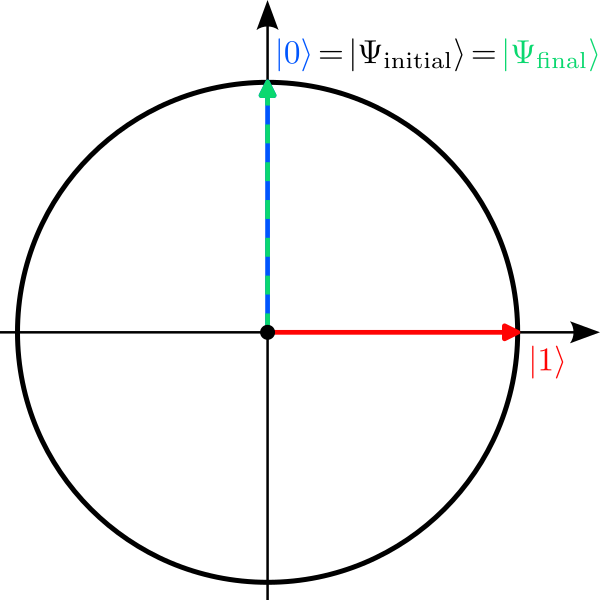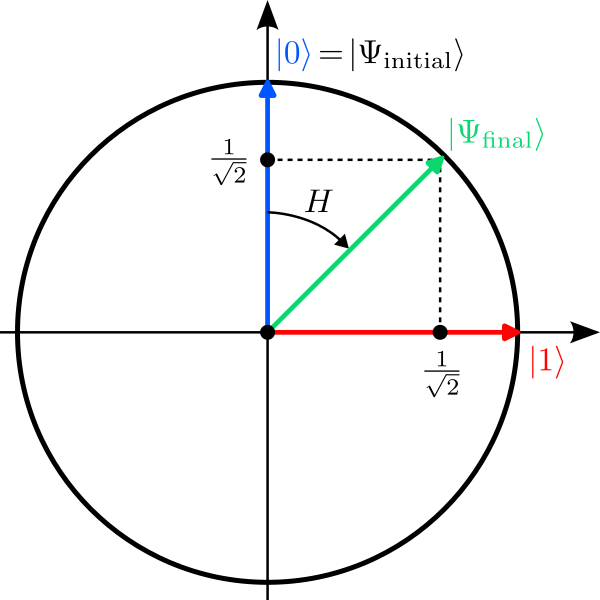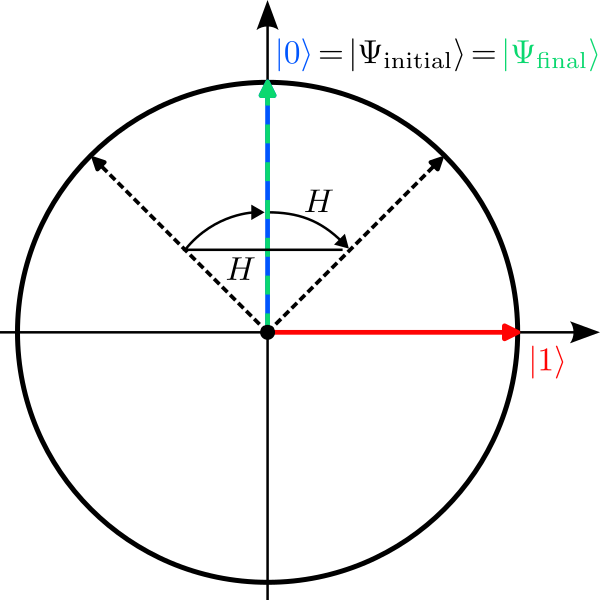You can use our interactive quantum circuit designer to run simple quantum programs on our servers which simulate a quantum computer (no account required!). To learn the basics of quantum mechanics, have a look at our tutorial.

Open the quantum circuit designer in a separate window.

Here a few suggestions how to use the quantum circuit designer to explore the features of quantum mechanics:

### How to use the quantum circuit designer?

We are assume that you are familiar with the basics of quantum mechanics.

TODO

• Bla

### Superpositions, Randomness, and Interference

For our first simulations, we use only a single qubit. In the circuit designer you should focus on the first line labeled q.

#### Simulation 1: Doing nothing

Let us start with the simplest quantum algorithm imaginable: The “do nothing algorithm”. It consists of the preparation of the qubit in the zero state $\ket{0}$ and its subsequent measurement, without any quantum gates in-between. As this is the default configuration of the circuit designer, you do not have to do anything, i.e., the circuit should look like this:

Run the simulation several times. Can you explain the observed probability distribution in the histogram? Do you find any randomness in the outcomes?

## Explanation

Without any gates, the transformation applied by the quantum computer is simply:
$$\ket{\Psi_\mathrm{initial}}=\ket{0} \;\xrightarrow{\; I \;}\; \underbrace{1}_{\alpha}\ket{0}+\underbrace{0}_{\beta}\ket{1} =\ket{\Psi_\mathrm{final}} \;\xrightarrow{\mathrm{Measure}}\; \begin{cases}\ket{0}&:\,p_0=1\\\ket{1}&:\,p_1=0\end{cases}$$Here $I$ denotes the “do nothing operation” called identity. At the end the final state is measured and yields the result $\ket{0}$ with probability $p_0=1$. So in this example, the measurement outcome is indeed deterministic (but also very boring).The qubit is initialized in the state $\ket{\Psi_\mathrm{initial}}=\ket{0}$ (blue) and ends up in the same state as final state $\ket{\Psi_\mathrm{final}}$ (green) since no gate is applied. This called the identity gate.

#### Simulation 2: Creating a superposition

Let us now create a superposition by applying a single Hadamard gate $H$ to our qubit. Its action on the two basis states of the qubit is defined as follows:\begin{aligned} \ket{0}\;&\xrightarrow{\;H\;}\;\frac{1}{\sqrt{2}}\ket{0}+\frac{1}{\sqrt{2}}\ket{1}\\ \ket{1}\;&\xrightarrow{\;H\;}\;\frac{1}{\sqrt{2}}\ket{0}-\frac{1}{\sqrt{2}}\ket{1} \end{aligned}Note that the vectors after the transformation have still length 1 since $\alpha^2+\beta^2=1$ in both cases! Geometrically, the Hadamard gate is the concatenation of two operations: A reflection about the y-axis followed by a clockwise 45° rotation about the origin.

The Hadamard gate is represented by a box with the label “H” in the circuit designer. Grab the box with your mouse and drop it onto the first line so that the gate is applied to the qubit with index 0. The circuit should look like this:

Run the simulation several times and observer how the results change with each run. Can you explain the observed probability distribution in the histogram?

## Explanation

Applying a Hadamard gate $H$ to the qubit creates a superposition:
$$\ket{\Psi_\mathrm{initial}}=\ket{0} \;\xrightarrow{\; H \;}\; \underbrace{\frac{1}{\sqrt{2}}}_{\alpha}\ket{0}+\underbrace{\frac{1}{\sqrt{2}}}_{\beta}\ket{1}=\ket{\Psi_\mathrm{final}} \;\xrightarrow{\mathrm{Measure}}\; \begin{cases}\ket{0}&:\,p_0=0.5\\\ket{1}&:\,p_1=0.5\end{cases}$$In this case, we wittness the randomness of quantum mechanics for the first time: The qubit is measured in the state $\ket{0}$ with probability $p_0=\alpha^2=0.5$ and in the state $\ket{1}$ with probability $p_1=\beta^2=0.5$. The result you get from our simulator is based on $N=200$ shots, so that you can expect roughly $N_0\approx 100$ shots where the results was $\ket{0}$ and $N_1=N-N_0\approx 100$ where it was $\ket{1}$.A Hadarmard gate describes a reflection about the y-axis followed by a clockwise rotation by 45°. The initial state $\ket{\Psi_\mathrm{initial}}=\ket{0}$ (blue) is therefore transformed into the final state $\ket{\Psi_\mathrm{final}}$ (green) which is a superposition of $\ket{0}$ and $\ket{1}$.

#### Simulation 3: Interference

One Hadamard gate creates a superposition with non-zero probability to find the qubit in the state $\ket{1}$. What happens if we apply a second Hadamard gate after the first one? Does the probability to find $\ket{1}$ increase further? Well, let’s try. Grab another “H” box and place it after the first one:

Run the simulation again several times and observer the histogram. Can you explain why the result is again deterministic and you never observe the state $\ket{1}$?

## Explanation

Applying two Hadamard gates $H$ transforms the qubit state as follows:
\begin{aligned} \ket{\Psi_\mathrm{initial}}=\ket{0} \;&\xrightarrow{\; H \;}\;\frac{1}{\sqrt{2}}\ket{0}+\frac{1}{\sqrt{2}}\ket{1} \\ &\;\xrightarrow{\; H \;}\; \frac{1}{\sqrt{2}}\left(\frac{1}{\sqrt{2}}\ket{0}+\frac{1}{\sqrt{2}}\ket{1}\right)+\frac{1}{\sqrt{2}}\left(\frac{1}{\sqrt{2}}\ket{0}-\frac{1}{\sqrt{2}}\ket{1}\right) \\ &\phantom{\;\xrightarrow{\; H \;}\;}= \underbrace{\left(\frac{1}{2}+\frac{1}{2}\right)}_{\alpha}\,\ket{0}+\underbrace{\left(\frac{1}{2}-\frac{1}{2}\right)}_{\beta}\,\ket{1} =\ket{\Psi_\mathrm{final}} \\ &\;\xrightarrow{\mathrm{Measure}}\; \begin{cases}\ket{0}&:\,p_0=1\\\ket{1}&:\,p_1=0\end{cases} \end{aligned}Here we had to use the rule how the Hadamard gate transforms the state $\ket{1}$. Note that the negative coefficient in this transformation precisely cancels the other term with $\ket{1}$, so that the final state has no contribution of $\ket{1}$ whatsoever. This cancelling of positive and negative amplitudes is called interference; it is one of the most important features for quantum algorithms. The final state after the application of two Hadamard gates is then just the initial state $\ket{\Psi_\mathrm{final}}=\ket{\Psi_\mathrm{initial}}=\ket{0}$ and we measure the qubit in state $\ket{0}$ with probability $p_0=1$.Applying the Hadarmard gate twice is equivalent to the identity gate. The initial state $\ket{\Psi_\mathrm{initial}}=\ket{0}$ (blue) is therefore the same as the final state $\ket{\Psi_\mathrm{final}}$ (green). This is an example of interference.

### Einstein’s “spooky action at a distance”: Entanglement

The phenomenon of entanglement is perhaps the most prominent feature of quantum mechanics. As it happens, it is also crucial for quantum computers to outperform their classical counterparts. Therefore it is not uncommon for larger quantum algorithms to start off with the preparation of entangled pairs (or even groups) of qubits. Here you will learn how this can be done with the quantum circuit designer and how entanglement is reflected in the measurement outcomes.

##### Simulation 1: Creating an entangled pair of qubits

Make sure that the circuit designer is setup with two qubits q and q.

To generate entanglement between two qubits we need two gates: The Hadamard gate $H$ (which operates on a single qubit), and the Controlled-NOT gate $\mathrm{CNOT}$ (which operates on a pair of qubits). The Hadamard gate has been discussed above in “Superpositions, Randomness, and Interference”. The Controlled-NOT gate flips one qubit (the target) depending on the state of another qubit (the control). Its action on quantum states is defined by its action on the four basis states of two qubits:
\begin{aligned} \ket{00}\;&\xrightarrow{\;\mathrm{CNOT}\;}\;\ket{00}\\ \ket{01}\;&\xrightarrow{\;\mathrm{CNOT}\;}\;\ket{01}\\ \ket{10}\;&\xrightarrow{\;\mathrm{CNOT}\;}\;\ket{11}\\ \ket{11}\;&\xrightarrow{\;\mathrm{CNOT}\;}\;\ket{10} \end{aligned}Note how the state of the second (target) qubit is flipped if and only if the state of the first qubit (control) is $\ket{1}$. In the circuit designer, the Controlled-NOT gate is depicted by a box with $\bullet$ and $\oplus$ connected by a line. The $\bullet$ signifies the control qubit, the $\oplus$ represents the target qubit.

To generate an entangled state, we first create a superposition state on the qubit q, and then use this qubit as the control of a Controlled-NOT gate with q as the target. To do this, drop a Hadamard gate and a Controlled-NOT gate in the circuit designer as follows:

Run this quantum circuit on the quantum simulator multiple times and observe the measurement outcomes. Can you theoretically explain the histogram? Can you explain with these results why entanglement cannot be used to send information faster than light?

## Explanation

The two gates transform the initial state as follows:
\begin{aligned} \ket{\Psi_\mathrm{initial}}=\ket{00} &\;\xrightarrow{\; H_0 \;}\; \frac{1}{\sqrt{2}}\ket{00}+\frac{1}{\sqrt{2}}\ket{10} \\ &\;\xrightarrow{\; \mathrm{CNOT}_{0\rightarrow 1} \;}\; \frac{1}{\sqrt{2}}\ket{00}+\frac{1}{\sqrt{2}}\ket{11} =\ket{\Psi_\mathrm{final}} \\ &\;\xrightarrow{\mathrm{Measure}}\; \begin{cases} \ket{00}&:\,p_0=0.5\\ \ket{01}&:\,p_1=0\\ \ket{10}&:\,p_2=0\\ \ket{11}&:\,p_3=0.5 \end{cases} \end{aligned}Here $H_0$ denotes a Hadamard gate applied to the qubit with index 0 and $\mathrm{CNOT}_{0\rightarrow 1}$ is a Controlled-NOT gate with control qubit 0 and target qubit 1. As a final result, one measures the states $\ket{00}$ and $\ket{11}$ with a probability $p_0=p_3=0.5$ each. The states $\ket{01}$ and $\ket{10}$ are never observed.

The state $\ket{\Psi_\mathrm{final}}=1/\sqrt{2}\left(\ket{00}+\ket{11}\right)$ is said to be entangled because on measuring the two qubits, it turns out that, while the outcome of one of them seems random, its partner always happens to choose the same value. This remains true even if the two qubits are separated by large distances after the Controlled-NOT gate has been applied, a phenomenon critized by Albert Einstein as a “spooky action at a distance“: If you look at one of the two qubits, it randomly decides to collapse into the state $\ket{0}$ or $\ket{1}$, but at the same time its partner seems to know about this random choice and collapses into the same state. Because quantum mechanics suggests that this happens instantaneously, it seems to violate the premise of Einstein’s theory of relativity that information cannot travel faster than light. This conclusion is false! The reason is that:

Correlation ≠ Information

• Entangled qubits can be arbitrarily far apart but one cannot use them to send information faster than light! In particular, there is no contradiction to Einstein’s theory of special relativity.
• Entanglement is used for quantum teleporation. Quantum teleportation does not teleport qubits; it transmits their state (maximally) at the speed of light.
• The creation of entanglement in the laboratory is standard in modern experiments — even in lab courses at universities.
##### Simulation 2: Creating many entangled qubits

Without any gates, the transformation applied by the quantum computer is simply:
$$\ket{\Psi_\mathrm{initial}}=\ket{0} \;\xrightarrow{\; I \;}\; \underbrace{1}_{\alpha}\ket{0}+\underbrace{0}_{\beta}\ket{1} =\ket{\Psi_\mathrm{final}} \;\xrightarrow{\mathrm{Measure}}\; \begin{cases}\ket{0}&:\,p_0=1\\\ket{1}&:\,p_1=0\end{cases}$$Here $I$ denotes the “do nothing operation” called identity. At the end the final state is measured and yields the result $\ket{0}$ with probability $p_0=1$. So in this example, the measurement outcome is indeed deterministic (but also very boring).

Make sure that the circuit designer is setup with three qubits q, q, and q.

Can you construct a circuit on more than three qubits that entangles all of them in a GHZ state? Assume there is a chance of $p=0.01$ (1%) for each qubit to be accidentaly measured in the timespan of a second (for instance, by the environment). What is the probability that a GHZ state with $N=10$ qubits collapses in one second? What is the probability for $N=100$ qubits?

## Explanation

Without any gates, the transformation applied by the quantum computer is simply:
$$\ket{\Psi_\mathrm{initial}}=\ket{0} \;\xrightarrow{\; I \;}\; \underbrace{1}_{\alpha}\ket{0}+\underbrace{0}_{\beta}\ket{1} =\ket{\Psi_\mathrm{final}} \;\xrightarrow{\mathrm{Measure}}\; \begin{cases}\ket{0}&:\,p_0=1\\\ket{1}&:\,p_1=0\end{cases}$$Here $I$ denotes the “do nothing operation” called identity. At the end the final state is measured and yields the result $\ket{0}$ with probability $p_0=1$. So in this example, the measurement outcome is indeed deterministic (but also very boring).

• GHZ states are important examples of quantum states that describe many entangled qubits.
• They can be used in experiments to probe the probabilistic nature of quantum mechanics.
• The more qubits are entangled in a GHZ state, the more likely it is that the state collapses.
##### Excursion: Schrödinger’s Cat

Without any gates, the transformation applied by the quantum computer is simply:
$$\ket{\Psi_\mathrm{initial}}=\ket{0} \;\xrightarrow{\; I \;}\; \underbrace{1}_{\alpha}\ket{0}+\underbrace{0}_{\beta}\ket{1} =\ket{\Psi_\mathrm{final}} \;\xrightarrow{\mathrm{Measure}}\; \begin{cases}\ket{0}&:\,p_0=1\\\ket{1}&:\,p_1=0\end{cases}$$Here $I$ denotes the “do nothing operation” called identity. At the end the final state is measured and yields the result $\ket{0}$ with probability $p_0=1$. So in this example, the measurement outcome is indeed deterministic (but also very boring).

Make sure that the circuit designer is setup with three qubits q, q, and q.

Can explain why in reality there is no superposition of a cat both being dead an alive?

## Explanation

Without any gates, the transformation applied by the quantum computer is simply:
$$\ket{\Psi_\mathrm{initial}}=\ket{0} \;\xrightarrow{\; I \;}\; \underbrace{1}_{\alpha}\ket{0}+\underbrace{0}_{\beta}\ket{1} =\ket{\Psi_\mathrm{final}} \;\xrightarrow{\mathrm{Measure}}\; \begin{cases}\ket{0}&:\,p_0=1\\\ket{1}&:\,p_1=0\end{cases}$$Here $I$ denotes the “do nothing operation” called identity. At the end the final state is measured and yields the result $\ket{0}$ with probability $p_0=1$. So in this example, the measurement outcome is indeed deterministic (but also very boring).

• The

### The No-Cloning Theorem and Quantum Error Correction

On a classical computer you can copy bits as much as you like. For instance, the bits that make up the content of this webpage have been copied to your computer from our server — it would be really stupid if they could not be copied but only moved because then only one person at a time could look at the webpage. Nonetheless, this is exactly how quantum information works! The mathematical fact that one cannot copy a qubit is known as No-Cloning Theorem.

##### The No-Cloning Theorem

Without any gates, the transformation applied by the quantum computer is simply:
$$\ket{\Psi_\mathrm{initial}}=\ket{0} \;\xrightarrow{\; I \;}\; \underbrace{1}_{\alpha}\ket{0}+\underbrace{0}_{\beta}\ket{1} =\ket{\Psi_\mathrm{final}} \;\xrightarrow{\mathrm{Measure}}\; \begin{cases}\ket{0}&:\,p_0=1.0\\\ket{1}&:\,p_1=0.0\end{cases}$$Here $I$ denotes the “do nothing operation” called identity. At the end the final state is measured and yields the result $\ket{0}$ with probability $p_0=1$. So in this example, the measurement outcome is indeed deterministic (but also very boring).

• The unknown quantum state of a qubits cannot be copied reliably.
• This No-Cloning Theorem follows from the linearity of quantum mechanics. It is therefore baked into the defining properties of quantum mechanics. The impossibility to clone quantum information is not a “downside to be fixed”.
• This is a problem for correcting errors on qubits.
• However, it can also be exploited for quantum cryptography.

### Your first quantum algorithm: The Deutsch-Jozsa Algorithm

For our first simulations, configure the simulator with a single qubit so that there is only one line in the quantum circuit.

##### Simulation 1: Doing nothing

Without any gates, the transformation applied by the quantum computer is simply:
$$\ket{\Psi_\mathrm{initial}}=\ket{0} \;\xrightarrow{\; I \;}\; \underbrace{1}_{\alpha}\ket{0}+\underbrace{0}_{\beta}\ket{1} =\ket{\Psi_\mathrm{final}} \;\xrightarrow{\mathrm{Measure}}\; \begin{cases}\ket{0}&:\,p_0=1.0\\\ket{1}&:\,p_1=0.0\end{cases}$$Here $I$ denotes the “do nothing operation” called identity. At the end the final state is measured and yields the result $\ket{0}$ with probability $p_0=1$. So in this example, the measurement outcome is indeed deterministic (but also very boring).

• A machine that follows the rules of quantum mechanics (a quantum computer) can solve certain problems much faster than a classical computer.
• We only know of a handful of problems where this applies (the most famous being integer factorization on which the RSA cryptosystem is based). Designing quantum algorithms is hard!

#### The Good

• Quantum computers can exponentially speedup the simulation of quantum mechanics itself!